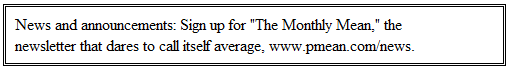P.Mean: How to report a one-tailed Fisher's Exact test (created 2008-07-12).Thank you for your informative page about the Fisher's Exact test. Can you please clarify how whether the test was 1 or 2-tailed affects the way that a significant result would be reported?

I love getting questions from people with good taste. By the way, I usually paraphrase these inquiries, but this is a direct quote.

First of all, I have to make a technical distinction.A one-tailed test uses a single tail of the probability distribution. The picture above shows a one-tailed test.A two-tailed test uses both tails of a probability distribution. The picture above shows a two-tailed test.A one-sided hypothesis examines changes in a single direction. The alternative hypothesis shown above is a one-sided hypothesis.A two-sided hypothesis examines changes in both directions. The alternative hypothesis shown above is a two-sided hypothesis.

For the t-test, a one-tailed test is used for a one-sided hypothesis and a two-tailed test is used for a two-sided hypothesis. This is also true for the Fisher's Exact test and many others. There are some situations, though, where you use a one-tailed test for a a two-sided hypothesis. The Chi-square distribution, for example, is used in some tests and usually (but not always) a single tail for the Chi-square distribution is sufficient for a two-sided hypothesis. This seemingly inconsistent result occurs because the Chi-squared distribution is frequently used with statistics that involve squaring. When you square a value, both extremely large negative and extremely large positive values end up in the right tail. the F distribution is another example of using a one-tailed test for a two-sided hypothesis.

I'm done with my technical distinction (some people would call it a nitpick). In general, many people use the term one-tailed test and one-sided hypothesis interchangeably, and I won't criticize anyone for this. Except for the poor person who sent me this inquiry and that nice compliment as well!

There are some technical issues with the actual calculation of a one-sided versus a two-sided Fisher's Exact test because the probability distribution isn't perfectly symmetric. but most people do not need to worry about this. I'm done with my technical nitpicks for today.

The key thing is to state clearly whether the test is one-tailed or two-tailed. This is usually done in the methods section. A statement like

All tests were conducted using a two-sided alpha level of 0.05

will work if you are using a two-sided hypothesis. If you are using a one-sided hypothesis, then you need to be a bit more careful. Something like

We used a one-sided hypothesis for the efficacy comparison, testing whether the new treatment was superior to placebo

works well.

You're also not allowed to change you mind about which test you used once the protocol is written and approved. That's considered a protocol deviation, and a pretty serious one at that. Generally, people will use nasty words like "fraud" and "cheating" if you change from a one-sided to a two-sided hypothesis after the data is collected. Changing from a two-sided to a one-sided hypothesis is equally bad. And when you get hauled before the Ethics board, I'll testify that anyone with the good sense to compliment my webpages deserves a break.

By the way, here's the R code that I used to draw the figures.

```x <- seq(0,20,by=0.1) y <- dchisq(x,df=5) a <- round(qchisq(0.025,df=5),1) b <- round(qchisq(0.975,df=5),1) par(mar=rep(0,4)) plot(x,y,type="n",axes=FALSE,xlab=" ",ylab=" ") polygon(c(x[x>=b],x[x==b]),c(y[x>=b],0),density=-1,border=NA,col="gray") lines(x,y) plot(x,y,type="n",axes=FALSE,xlab=" ",ylab=" ") polygon(c(x[x>=b],x[x==b]),c(y[x>=b],0),density=-1,border=NA,col="gray") polygon(c(x[x<=a],x[x==a]),c(y[x<=a],0),density=-1,border=NA,col="gray") lines(x,y)```This work is licensed under a Creative Commons Attribution 3.0 United States License. This page was written by Steve Simon and was last modified on 2010-04-01. Need more information? I have a page with general help resources. You can also browse for pages similar to this one at Category: Hypothesis testing, Category: Writing research papers.